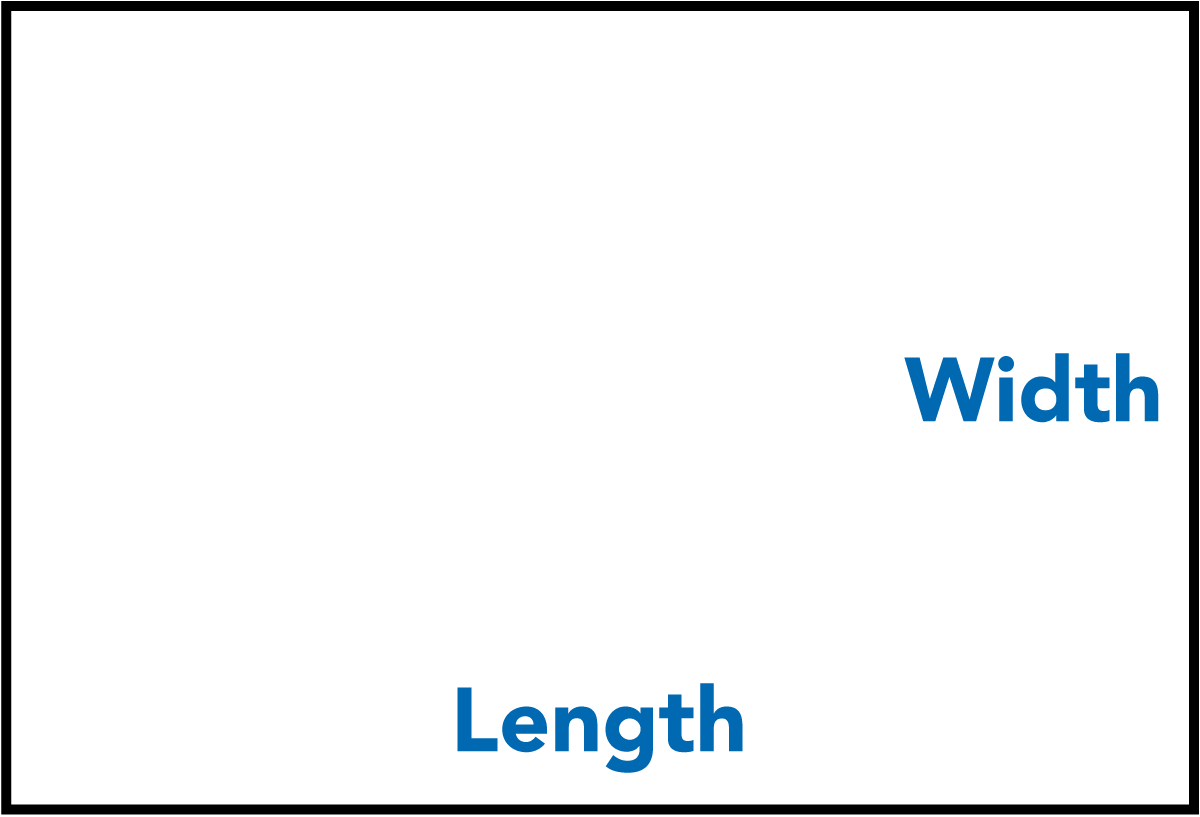# Square Metre Calculator - How To Calculate An Area - Australia

This free calculator will help you find out the area or number of square metres for a property, a piece of land, a room or a building project.

Learn how to use this calculator below!

### How do you calculate square metres?

First, you will need to think about the area you are trying to measure.

Most properties have multiple rooms, usually square or rectangular.

If so, you must split up these areas individually, calculate for each area separately and then add up all the results at the end.

Measure each area and enter your results into the calculator. Ideally, you should use a measuring tape to do this. Make sure to round up your measurements as necessary.#### For a rectangle or square:

Simply measure the length and the width.#### For a circle:

You will need to measure the middle of the circle by dividing it in half. This is called the diameter. Then half the diameter to get the radius, which you then input into the calculator above.

See all of our real estate and property calculators here.

We have calculators for working out commission, rent, salaries, fees, measurements and much more!